## Gallery

Some examples of the beauty of lattice models. These are just a select few I've come across when studying the phenomena involved. Behind every one is some theory, perhaps even a paper explaining it all. Follow the Publications link if you care.Above are two evolutions of 1d cellular automata (CA). Initial state is at the top and time runs downwards. The boundary conditions are periodic (left and right edges coincide). All the randomness is in the initial state - the CA rule itself is deterministic. Yet the left is essentially the same as the stochastic dynamics for the zero temperature Ising model (the Voter model) and the one on the right equates with positive temperature Ising dynamics. The "fine print" inside the three shades is where the randomness is carried on (stationarily) with an additive subCA. These are examples of partially permutive CA rules.A dynamic phase transition. The system is the 2d Golden mean or the Hard Square model. The left and right pictures represent the locations of 1 by 1 plaques (the hard squares) on even and odd lattices respectively. The configurations are generated with a probabilistic cellular automaton. When the rule finds four adjacent squares without a plaque in 2 by 2 formation centered on the even/odd lattice, it places with probability p a plaque on the odd/even lattice site at the joint corner of the quadruple. Notice that if you superimpose the images the blacks representing the plaques will not overlap - this is just the exclusion rule that the PCA implements. The critical p is around .79 - beyond that the generic packing of the plaques is the one which favors one of the lattices. Above p is .8125.

Below is a more subtle version of the splitting. Its still square lattice underneath but the neighborhood is Moore's (eight nearest neighbors). In the supercritical state illustrated the system has made a decision to pack the 1's (tiny dark squares) contiguously on rows rather than on columns. The remaining fight as the system relaxes to its equilibrium is about even or odd horizontal rows dominate (below left and right correspondingly).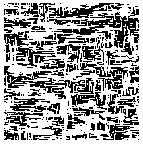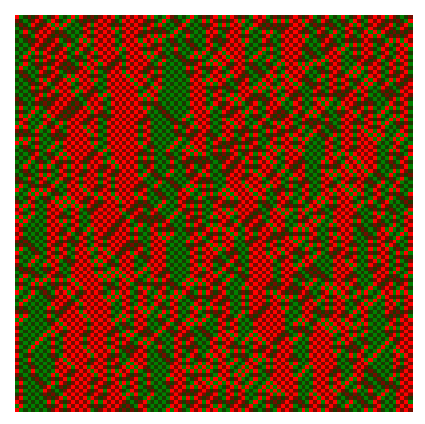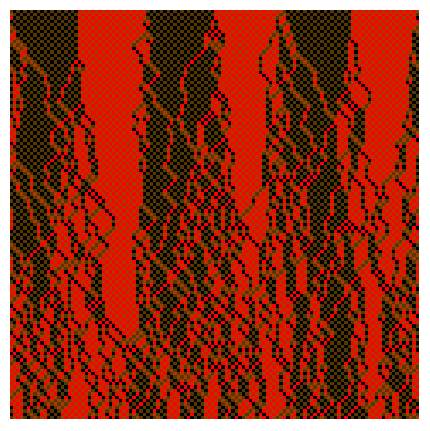The colored examples above are what you get when you generate the 2d Ice model configurations with a 1d PCA. Nasu called certain symbolic dynamics "textile systems" - not so far off, is it?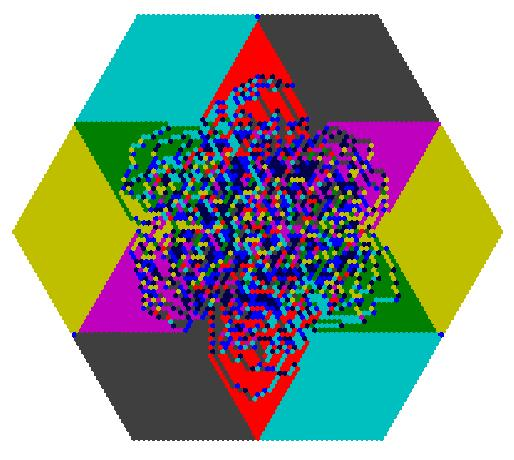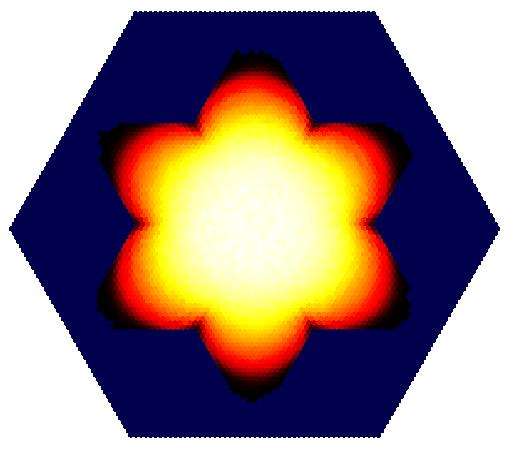The pics above illustrate the Arctic Circle phenomenon on the triangular lattice. This in particular relates to the Ice Model of Statistical Mechanics when confined to a hexagon. The boundary arrows satisfying certain height properties impose strong long range order to the interior of the configuration. The pic on the left is a representation of a generic configuration while the one on the right shows the density of local moves at the equilibrium of the cellular automaton generating the configurations. Navy blue means no local moves took place - the arrows on the boundary of the hexagon fix the configuration a macroscopic distance into the interior. The white zone is hottest i.e. where most of the configuration variety is generated. So the demarcation between frozen and temperate worlds isn't along a circle anymore - but then again even at the real Arctic Circle flowers do grow!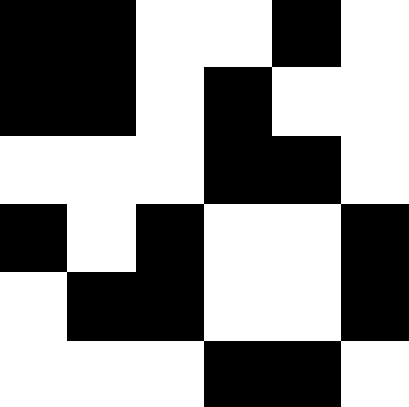Apart from fine textiles and flowers, elegant rooms often feature frieze patterns on the walls, right? Perhaps such as the strip above. Consider the following construction: fix k, an integer between 1 and 8. Distribute 0's and 1's on the square lattice so that in every 3 by 3 window there are exactly k 1's. If k=1, 2 or 3 (or 6, 7 or 8 by reflection) the sets of all corresponding configurations are each slide connected: by moving a row or column of period three we can transform one configuration to an other and by a finite sequence of such moves any legal configuration to another. If k=4 (or 5) this is not true! Using the 6 by 6 prototile (white is 0, black is 1)in a doubly infinite array to construct a tiling of the plane we have a configuration, each row and column of which is of period six. Hence it cannot be slide deformed. Tiles like this are very rare among the 6 by 6 legal tiles. The strip is just a cut from this exotic configuration.